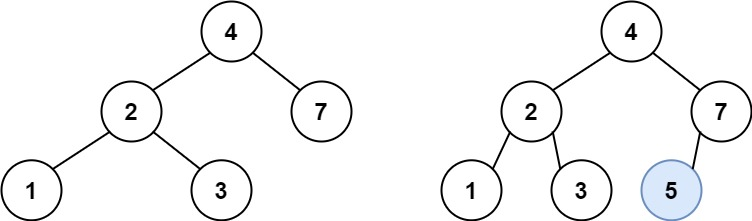# Insertion in Binary Search Tree

## Introduction

Binary search tree T is one type of binary tree that is either empty, or each node in the tree contains a key and:

(i) all keys in the left subtree of T are less (numerically or alphabetically) than the key  in the root node of T;

(ii) all keys in the right subtree of T are greater than the key in the root node of the BST;

(iii) the left and right subtrees of the BST are also binary search trees.

While inserting nodes in a binary search tree, we should be conscious that it should not violate the property of a binary search tree after insertion.

In this article, we will discuss different methods of inserting in BSTs.

## Problem Statement

You are given a binary search tree and a value(key) to insert into the tree. Return root of the BST after insertion. It is given that the new value does not exist in the given BST because binary search tr̥ees can not contain duplicate keys.

Input: keys[] = {4,2,7,1,3} value=5

Output: 1 2 3 4 5 7 (inorder)Source: LeetCode

In the above picture,  the node with a value  5 would be inserted in the right subtree of the root node, i.e., 4, and as the left child of node 7 as 5 < 7 and 4 < 5.

## Method 1: Iterative method

### Algorithm

• For inserting in the Binary Tree, the value is compared with that of the root.
• If the given value is lesser than the root’s, then move to root’s left, and it is compared with root’s left child value.
• If the given value is greater than the root’s, then move to root’s right, and it is compared with root’s right child value.
• This comparison continues until a leaf node is reached.
• Then new node is inserted as a left or right child of the leaf node depending on its value.
• For empty BST, the value is directly inserted as the root’s key.

### Complexity Analysis

Time complexity: For the above solution, the time complexity is O(h), where h is the height of the binary search tree. In the worst-case height becomes equal to the number of nodes in the BST(skewed tree).

Space complexity: O(1)

## Method 2 : Recursive method

### Algorithm

• (Base Condition) Examine the root if it is null, create BST node with given value and return the node pointer
• If the given value is lesser than the root’s, recursively insert the new node to the left subtree.
• If the given value is greater than the root’s, recursively insert the new node to the right subtree.

### Complexity Analysis

Time complexity: For the above solution, the time complexity is O(h), where h is the height of the binary search tree. In the worst-case, height becomes equal to the number of nodes in the BST(skewed tree).

Space complexity: Due to stack space for recursive calls, space complexity is O(h) at worst case.

## FAQs

1. What is a Binary Tree?
A tree is called a binary tree if every node has at most two children nodes.  A binary tree node constitutes a left pointer, a right pointer, and a data element. An empty binary tree (with zero nodes) is also a valid binary tree.

2. What are Binary Search Trees?
A binary search tree T is a specific type of binary tree that is either empty, or each node in the tree contains a key and
(i) all keys in the left subtree of T are less (numerically or alphabetically) than the  identifier in the root node of T;
(ii) all keys in the right subtree of T are greater than the identifier in the root node of T;
(iii) the left and right subtrees of T are also binary search trees

3. Why are Binary Search Trees used?
(i)Time complexity on insertion, deletion, and searching in binary search trees gets reduced as it works on the principle of binary search rather than linear search, as in typical binary trees.
(ii)Self-balancing binary search trees(like AVL trees) are used to implement priority queues.
(iii)They are used to implement tree sets and hashmaps.
(iv)In various programming languages, BSTs are used to implement dictionaries.
(v)Multi-level indexing in Databases is implemented using binary search trees.

4. What are the different scenarios that take place when inserting nodes in a Binary Search Tree?
During insertion in Binary Search Tree, it can be an empty binary tree or not. If it is empty, the new node will be considered the root node; otherwise, we need to search the position for inserting the new node in the Binary Search Tree.

5. Describe the time and space complexities of making insertions in a binary search tree.
In general, the time complexity of making insertions in a binary search is O(h), where h is the height of the BST.
For interactive approach, space complexity for inserting in BSTs is O(1)

## Key Takeaways

This article covered different methods of how to make insertion in the Binary Search Tree. We have learned about the various algorithms to insert nodes in BSTs, but that’s not enough for us.

Side by side, we should also learn about searching and deletion operations in  Binary Search Tree.

Apart from this, you can practice a wide range of coding questions commonly asked in interviews in CodeStudio. Along with coding questions, we can also find the interview experience of scholars working in renowned product-based companies here.
After you've mastered insertion, you should learn how to delete a node in BST. Check out the video below, which covers both the insertion and deletion of a node in BST.

Happy learning!